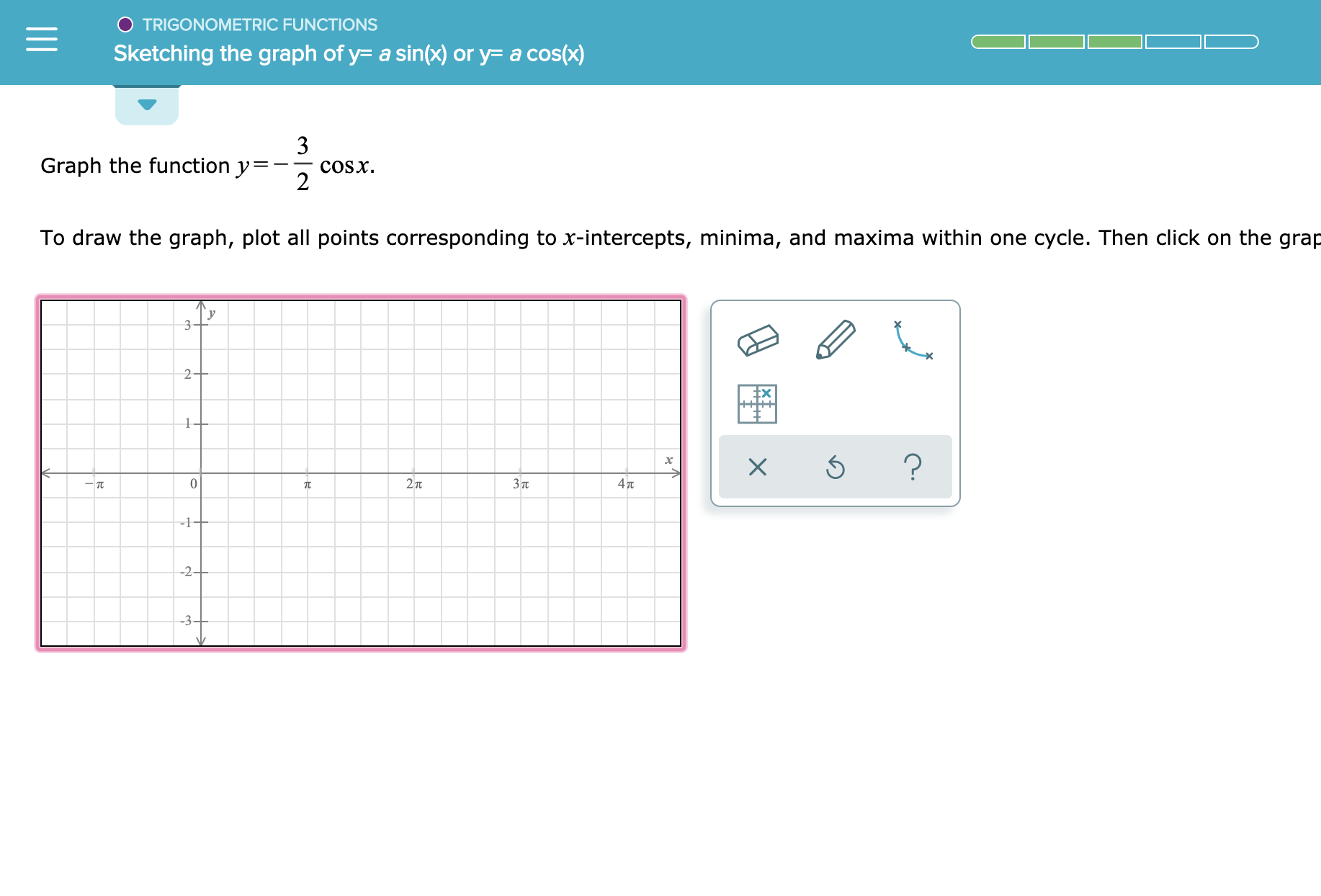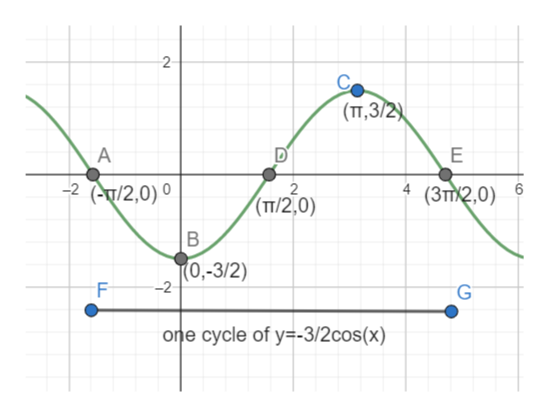# TRIGONOMETRIC FUNCTIONSSketching the graph of y- a sin(x) or y= a cos(x)3COSX2Graph the function y=To draw the graph, plot all points corresponding to x-intercepts, minima, and maxima within one cycle. Then click on the grap32+102TЗп47t-1--2+X

Question
3 views

See attachmenthelp_outlineImage TranscriptioncloseTRIGONOMETRIC FUNCTIONS Sketching the graph of y- a sin(x) or y= a cos(x) 3 COSX 2 Graph the function y= To draw the graph, plot all points corresponding to x-intercepts, minima, and maxima within one cycle. Then click on the grap 3 2+ 1 0 2T Зп 47t -1- -2+ X fullscreen
check_circle

The given function is y=-3/2cos(x). As cos(x) is periodic function with fundamental period 2pi. The range of the functi...help_outlineImage Transcriptionclose2 C (TT,3/2 A E -2 /2,0) 2 (3TT/2,0) (TT/2,0) 0,-3/2) -2 G one cycle of y-3/2cos(x) CO m L fullscreen

### Want to see the full answer?

See Solution

#### Want to see this answer and more?

Solutions are written by subject experts who are available 24/7. Questions are typically answered within 1 hour.*

See Solution
*Response times may vary by subject and question.
Tagged in

### Calculus